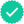Question

A and B are independent events. Use the following probabilities to answer the question. Round to 4 decimal places.
P(A) = 0.19, and P (A and B ) =0.05 , find P(B)

Expert SolutionKnowledge Booster••